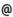## "Babes-Bolyai" University of Cluj-Napoca Faculty of Mathematics and Computer Science

 Dynamical Systems
 Code Semes-ter Hours: C+S+L Type Section MME0002 2 2+1+1 compulsory Informatică
 Teaching Staff in Charge
 Prof. TRIF Damian, Ph.D.,  dtrifmath.ubbcluj.ro
 Aims Introduction to the basic problems of dynamical systems and mathematical modeling
 Content Introduction (basic terminology, examples, computers and dynamical systems) Unit I: Discrete and continuous dynamical systems (recurrences and ordinary differential equations as dynamical systems, first order equations, higher order differential equations, existence theorems, general linear ordinary differential equations, boundary value problems) Unit II: Qualitative Analysis (limit sets, singular points, stability theory, invariant sets, Poincaré maps, bifurcation theory) Unit III: Numerical Approach (approximate solutions, numerical stability, continuation, calculation of the bifurcation behavior, chaos)
 References Davis Jon H., Differential Equations with MAPLE: an Interactive Approach, Birkhäuser, 2001. Enns R. H., McGuire G., Nonlinear Physics with Maple for Scientists and Engineers, Birkhäuser, 1997. Hairer E., Numerical Geometric Integration, Internet course, 1999, http://www.unige.ch/math/folks/hairer/polycop.html Lynch S., Dynamical Systems with Applications using MATLAB, Birkhäuser, 2004. Rus I.A., Ecuatii diferentiale, ecuatii integrale si sisteme dinamice, Transilvania Press, 1996. Trif D., Metode numerice in teoria sistemelor dinamice, Transilvania Press, 1997.
 Assessment 1.Two in-class midterm exams, each worth 20% of course 2.Lab report, worth 10% of course grade. 3.Final exam, worth 50% of course grade.
 Links: Syllabus for all subjects Romanian version for this subject Rtf format for this subject